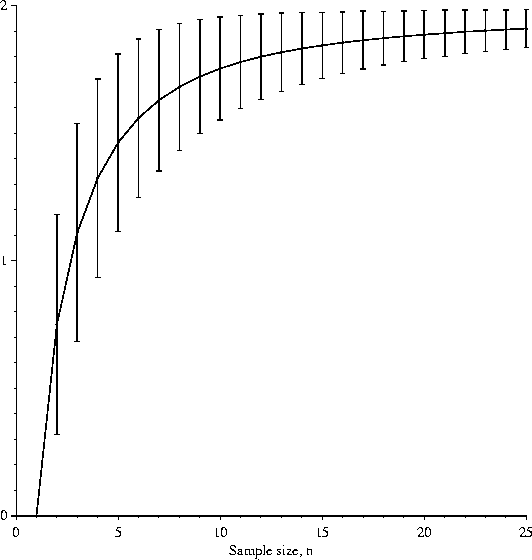>Next: (d) Variance of the Up: APPENDIX Previous: (b) Approximate method

## (c) Use of the Correction Factor

The two methods of calculation produce the expected uncertainty of nsample bases, E(Hnb):

 E(Hnb) =  Hg - e(n)       (bits per base). (17)

When Hs(L) is calculated from a small sample, it is too small by the amount e(n), on the average. To correct Rsequence(L), we use:

 Rsequence(L)  =  Hg  -  [Hs(L)  +  e(n)]       (bits per base). (18)

That is, the uncertainty of the pattern is increased because there is only a small sample. Substituting equations (17) and (18) into (5) gives equation (6). Hg could also be corrected but the correction is negligible if Hg is calculated from a large sample of the organism's sequence.

The curve for E(Hnb) as a function of the number of example sites, n, (Fig. 5) has several important general properties. As the number of example sites increases, E(Hnb) approaches Hg(= 2 bits/base in the figures) since the error e(n) becomes smaller. As the number of examples drops, E(Hnb) also drops (the error increases), until at only one example E(Hnb) is zero. With only one example, the uncertainty of what the sequence is, Hs(L), is also zero. At this point, Rsequence is forced to zero (from equation 6): one cannot measure an information content from only one example.These data are for an equiprobable genomic composition. The curve is less than 1% lower for the composition of E. coli. Each bar represents one standard deviation above and below the curve.

The sampling error correction results in an interesting effect. If Rsequence could be measured for an infinite number of HincII sites (this would look something like Fig. 1a), the two peaks would be 2 bits/base. When the correction is made for a small sample, the peaks are less than 2 bits/base (Figs. 1b and 1c). This appears odd if we know exactly what HincII recognizes. However, given only six examples, we would not be so sure what the "real" pattern is. The sampling error correction prevents us from assuming that we have more knowledge than can be obtained from the sequences alone. That is, the value e(n) represents our uncertainty of the pattern, owing to a small sample size. In the extreme case of one sequence, we have no knowledge of what the pattern at the site is, even though we see a sequence. Because of the correction, Rsequence will be underestimated at truly conserved positions when only a few sites are known. Rsequence for six HincII sites in Fig. 1c is estimated to be 8 bits even though we "know" (by looking at more than six examples) that HincII recognizes 10 bits.Next: (d) Variance of the Up: APPENDIX Previous: (b) Approximate method
Tom Schneider
2002-10-16